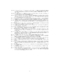## Maintaining Directed Reachability with Few Edges##### Files
CS-TR-3134.ps(312.39 KB)
The MEG (minimum equivalent graph) problem is the following: Given a directed graph, find a smallest subset of the edges that maintains all reachability relations between nodes.'' This problem is NP-hard; this paper gives an approximation algorithm achieving a performance guarantee of about 1.64 in polynomial time. The algorithm achieves a performance guarantee of 1.75 in the time required for transitive closure. The heart of the MEG problem is the minimum SCSS (strongly connected spanning subgraph) problem --- the MEG problem restricted to strongly connected digraphs. For the minimum SCSS problem, the paper gives a practical, nearly linear-time implementation achieving a performance guarantee of 1.75. The algorithm and its analysis are based on the simple idea of contracting long cycles. The analysis applies directly to 2-Exchange, a general local improvement'' algorithm, showing that its performance guarantee is 1.75. (Also cross-referenced as UMIACS-TR-93-87)